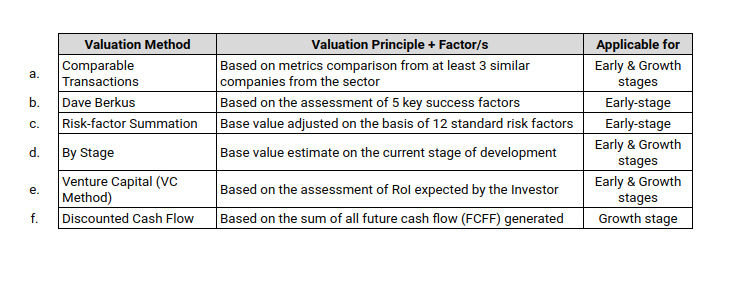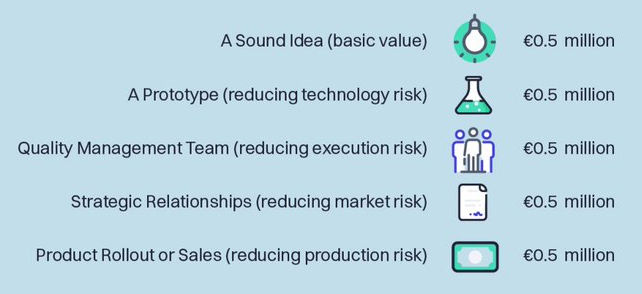top of page
Search

# How to Calculate the Fair Valuation of Your Startup?

Startup valuation is complicated, and not surprisingly, many entrepreneurs struggle hard with "calculating startup valuation?" More so with the pre-revenue startups that have none, or barely little traction to prove validation.Once the startups have steady revenues and earnings, then the valuation is pretty simple i.e. valuing them as a multiple of their EBITDA and/or industry-specific PE multiples based on the comparable data that is readily available.

In this article, we'll delve into various startup valuation methods, which you can use to estimate the value of your startup.

## What is a Valuation?

Valuation is the process of quantifying the worth of a business, simply put how much is it worth at any given point in time. During funding rounds, investors fund a startup in exchange for a part of the equity in the business.

From a fundraising perspective, startup valuation is essential as it helps in determining the equity portion, which they have to give away to an investor in return for their funding.

The 2 key elements of valuation are

### 1. Pre-money Valuation

For an investor, the first step is to agree on the Pre-money valuation; i.e., what the startup is worth before they raise any external funding.

### 2. Post-money Valuation

Post-money valuation is calculated as the worth of a business after an investment has been made. This can be best depicted as the below formula:

• Post-money Valuation = Pre-money Valuation + Investment (by the Investor)Basis the given example, with a \$250K investment, the investor would end up having a 25% stake in the business.

## Why is Valuation Important for Startups?

The valuation of a startup is one of the most important things to know when fundraising, as it helps determine the fair amount of equity they have to give to an investor in exchange for funds. It requires a fine balance so as to not undervalue or overvalue your startup.

• Investors would want to calculate their potential return on their investment (IRR).

• Founders don't want to give away too much equity in return for funding, especially at the early stages.

"Nothing turns off an investor more than when an entrepreneur comes in with a ridiculous valuation" - Kevin Harrington

## Startups Valuation Methods

Although there are many startup valuation methods, in this article we'll cover the 6 most commonly used ones that investors usually follow for valuing startups, before issuing the term sheet.

Here's the snapshot of these 6 startup valuation methods:Let's now define each of them ...

### a. Comparable TransactionsVenture Capitalists usually like this approach of comparable transactions, as it gives them a pretty good indication of what the market is willing to pay for a company.

The comparable transactions method values a startup by determining how many other similar startups were acquired in recent years and uses that information as a precedent to arrive at an appropriate value range. This method may work best if you are comparing two startups offering similar products or comparable services.

### b. Dave Berkus Valuation Method

The Berkus Method of Valuation is a prominent early-stage valuation method.It was explicitly created to find a starting point without relying upon the founder’s financial forecasts. Berkus Method studies 5 crucial stages of a startup and indicates a value ranging from \$0 to \$500,000 for each stage.

### c. Risk-Factor Summation Method

Risk-Factor Summation (RFS) is a rough pre-money valuation method for early-stage startups.In this method, you’ll evaluate a startup based on 12 risk factors (i.e. the stage of business, management risk, manufacturing risk, legislation/political risk, sales and marketing risk, funding and capital raising risk, competition risk, technology risk, litigation risk, international risk, reputation risk, and a potentially lucrative exit) to determine its pre-money valuation.

Less risk, more value. High risk, less value. You will need to add/subtract (\$0 to \$500,000) from the bottom line based on those scores.

### d. Valuation by StageThen there is the development stage valuation approach, often used by investors to quickly calculate a rough estimate of company value. Such "rule of thumb" values are typically set by the investors, based on the venture's current stage of development.

For example, a startup at the ideation (business plan) stage will likely get the lowest valuation, while once achieving some early traction can demand a higher value. The ranges might vary depending on the company and the investors.

### e. Venture Capital Valuation MethodThe Venture Capital Valuation methodology is simple and derived from the below equations:

ROI = Terminal value / Post-money Valuation, where Terminal value is the anticipated selling price for the startup in the future (i.e. usually 5 to 8 years for early-stage.)

It can be estimated by establishing a reasonable expectation for revenues in the year of sale and, based on those revenues, estimating earnings in the year of the sale.

For example, we believe a startup could be acquired for \$20 Mn in a few years, and we expect to receive a 10X return on our investment.

Using the formula, 10X = \$ 20 Mn / Post-money Valuation

Post-money Valuation = \$ 20 Mn / 10 = \$ 2 Mn

Let's say we would like to invest \$ 200K in this venture, the Pre-money Valuation = \$ 2 Mn - \$ 200K = \$ 1.8 Mn, and the required equity stake would be 10% (\$ 200K/\$ 2 Mn)

### f. Discounted Cash Flow (DCF) Method

This method relies on market analysis to make predictions about the company's future growth and how that may affect its overall profits.DCF involves forecasting how much cash flow the company will produce in the future (FCFF) and then, using an expected rate of investment return, calculating how much that cash flow is worth. DCF analysis thus attempts to determine the value of an investment today, based on projections of how much money that investment will generate in the future.

A higher discount rate is typically applied to startups, as there is a high risk that the company will inevitably fail to generate sustainable cash flows.

### Good Practice

Try using the average of at least 3 valuation methods to estimate the appropriate pre-money valuation for a startup.

## Valuations are Declining Q-o-Q in 2023As per Angellist, the average startup valuation has declined in Q3-2023 from Q2-2023 at every stage, except for Pre-seed.

• Pre-seed: valuations rose by 8% to \$10M.

• Seed: valuations declined by 8.4% to \$21.7M.

• Series A: valuations declined by 8.4% to \$76.7M.

• Series B: valuations declined by 2.6% to \$216M.

The above data may prove the hypothesis that VCs are re-entering the market due to the lower cost of investment.

## Summary

So to summarize:

• Valuation is a highly complex task for ventures that have little to no revenue.

• By valuing a startup, the founders determine the fair amount of equity they've to give to the investor in exchange for their funds.

• Post-money Valuation = Pre-money Valuation + Investment.

• There are 6 commonly used methods for calculating startup valuation:

1. VCs often use the Comparable Transactions approach to get a pretty fair indication of what the market is willing to pay for a company.

2. Dave Berkus is a prominent early-stage valuation method that uses a value (ranging from \$0 to \$500K) for each of the 5 crucial stages of a startup to arrive at a rough valuation.

3. Risk-Factor Summation (RFS) is a rough pre-money valuation for early-stage startups.

4. The Valuation by Stage approach is often used by investors to quickly calculate a rough estimate of company value.

5. The Venture Capital Valuation methodology is simple and derived from the equation: ROI = Terminal value / Post-money Valuation, where Terminal value is the anticipated selling price for the startup in the future

6. Discounted Cash Flow (DCF) refers to a valuation method that estimates the value of an investment using its expected future cash flows.

• A good practice is to use the average of at least 3 valuation methods to estimate the appropriate Pre-money valuation for a startup.

Related Articles: